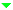Optical Materials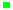Optical GlassLow Expansion MaterialsIR MaterialsCrystals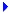Micro-Optical ComponentsFiber Optic ComponentsLight Sources & PhotodiodesWafersServices

Refractive Index, Abbe Value and Dispersion Formula

The optical properties are defined by two primary parameters, the refractive index and Abbe number. The refractive index is a measure of the bending power of a light beam when passing from one medium into another. The Abbe or v-number named after German physicist Ernst Abbe, is a measure of the dispersive power and is defined as: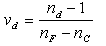(1)

Where nd, nF and nC are the refractive indices of the material at wavelengths of the Fraunhofer d-, F- and C- spectral lines (587.6 nm, 486.1 nm and 656.3 nm respectively). Besides , the dispersion based on e-line is often used as: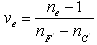(2)
 Glass Type nd Vd Density (g/cm3) Glass Code N-BK7 1.51680 64.17 2.51 517642 N-SF11 1.78472 25.68 3.22 785257 Table 1: Examples of glass code and primary parameters

Table 1 shows some basic information of glass code, two primary optical parameters and density. Table 2 shows spectral lines and designated letters for them. The refractive indices of optical glasses are given at these wavelengths with the N-BK7 as an example.

 Wavelength (nm) Designated Letter Element N-BK7 Refractive Indices Spectral Line 1013.98 t Hg 1.50731 Infrared mercury line 852.11 S Cs 1.50980 Infrared cesium line 706.52 r He 1.51289 Red helium line 656.27 C H 1.51432 Red hydrogen line 643.85 C' Cd 1.51472 Red cadmium line 632.8 632.8 He-Ne Laser 1.51509 Helium-neon gas laser 589.29 D Na 1.51673 Yellow sodium line      (center of the  double line) 587.56 d He 1.51680 Yellow helium line 546.07 e Hg 1.51872 Green mercury line 486.13 F H 1.52238 Blue hydrogen line 479.99 F' Cd 1.52283 Blue cadmium line 435.83 g Hg 1.52668 Blue mercury line 404.66 h Hg 1.53024 Violet mercury line 365.01 i Hg 1.53627 Ul traviolet mercury line Table 2: Wavelengths used for spectral lines

The refractive index at a wavelength other than the spectral lines can be calculated from a dispersion formula. SCHOTT uses Sellmeier dispersion formula: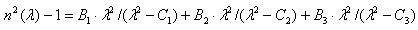(3)

where λ is the wavelength in um ,and B1, B2, B3, C1, C2 and C3 are coefficients to be determined for each optical glass type. Table 3 gives the parameters of N-BK7 and N-SF11. HOYA’s dispersion formula is derived from a series expansion of the theoretical formula: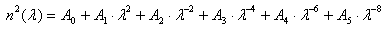(4)

where λ is the wavelength in um ,and A0, A1, A2, A3, A4 and A5 are coefficients. The accuracy of both formulas for calculating refractive index at a wavelength in the visible and near infrared range has an order of 10-6.

 Constants of dispersion B1 B2 B3 C1 C2 C3 N-BK7 1.03961212 0.231792344 1.01046945 0.00600089867 0.0200179144 103.560653 N-SF11 1.73759695 0.313747346 1.89878101 0.013188707 0.0623068142 155.23629 Table 3: Constants of dispersion parameters of N-BK7 and N-SF11

Properties: